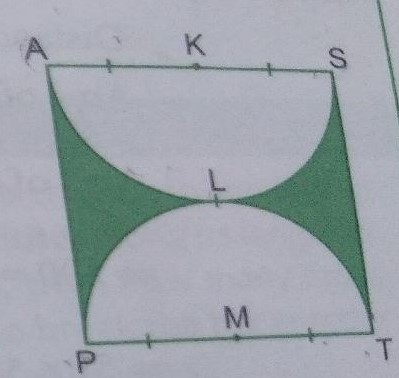"
">

# Find the area of the shaded region if PAST is square of side 14 cm and ALS and PLT are semicircles."

Given:
PAST is square of side 14 cm and ALS and PLT are semicircles.
To do:
We have to find the area of the shaded region.
Solution:
Here, as given in the above question $PAST$ is a square and $ALS$ and $PLT$ are two semi-circles.
$\because PAST$ is a square.
$\because$  Side of the square PAST is 14 cm.
$\therefore PA=AS=ST=TP=14\ cm$.
Here, PT and AS are diameters of semi-circles PLT and ALS.
$\therefore$ Radius of the semi-circles PLT and ALS $=\frac{14}{2}\ cm=7\ cm$.
Therefore,
Area of square PAST$=(14)^2\ cm^2=196\ cm^2$.
Area of semicircle PLT$=\frac{22}{7}\times\frac{7^2}{2}\ cm^2=11\times7\ cm^2=77\ cm^2$.
Area of semicircle ALS$=\frac{22}{7}\times\frac{7^2}{2}\ cm^2=11\times7\ cm^2=77\ cm^2$.
Area of the shaded region$=$Area of the square PAST$-$(Sum of the areas of semicircle PLT and ALS)
$=196-(77+77)\ cm^2$
$=196-154\ cm^2$
$=42\ cm^2$
The area of the shaded region is $42\ cm^2$.

Updated on: 10-Oct-2022

278 Views